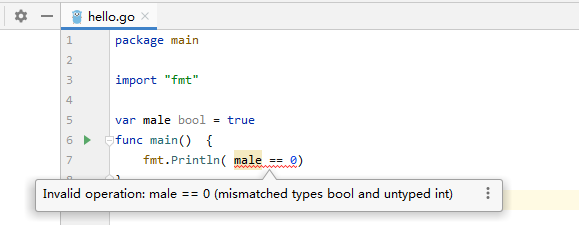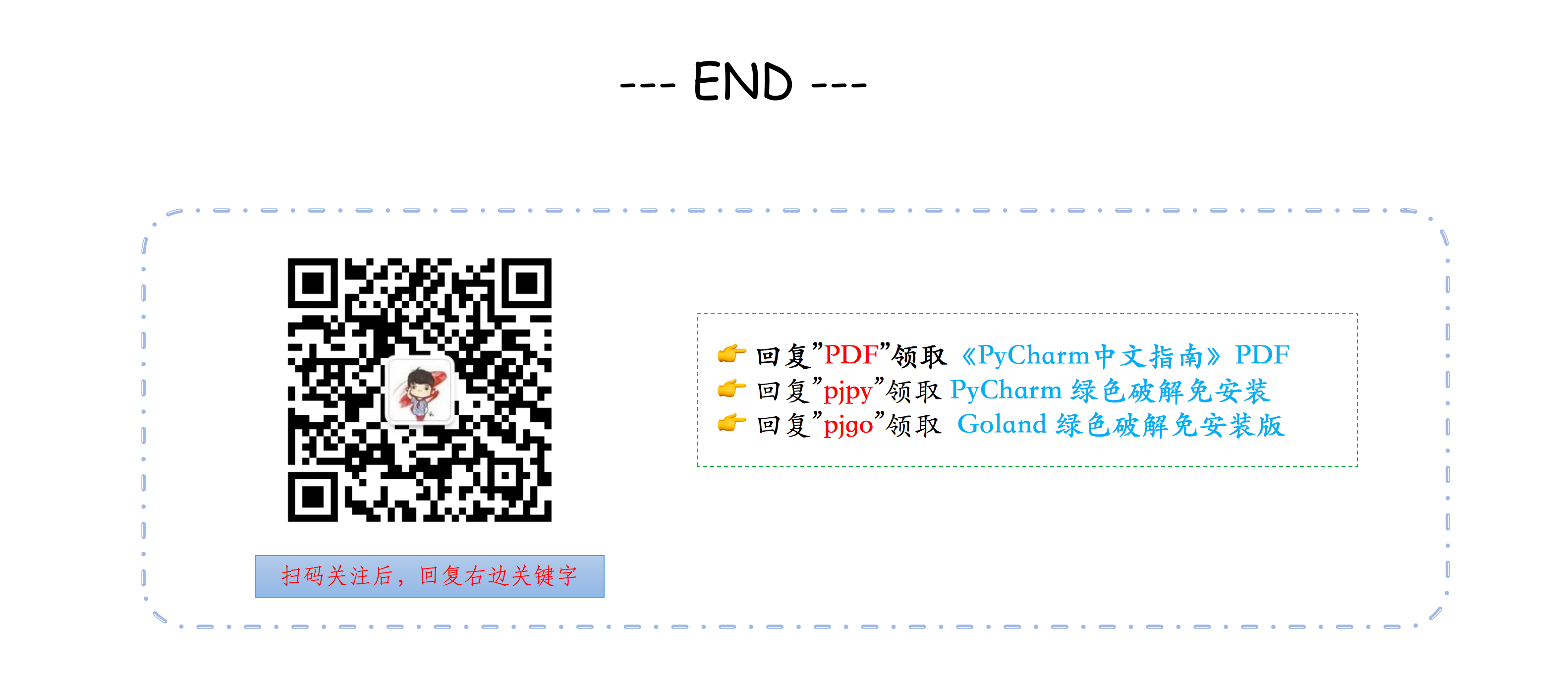# 零基础学 Go 语言（06）：数据类型之字典与布尔类型

## 1. 字典

map[KEY_TYPE]VALUE_TYPE


### 声明初始化字典

// 第一种方法
var scores map[string]int = map[string]int{"english": 80, "chinese": 85}

// 第二种方法
scores := map[string]int{"english": 80, "chinese": 85}

// 第三种方法
scores := make(map[string]int)
scores["english"] = 80
scores["chinese"] = 85


import "fmt"

func main() {
// 声明一个名为 score 的字典
var scores map[string]int

// 未初始化的 score 的零值为nil，无法直接进行赋值
if scores == nil {
// 需要使用 make 函数先对其初始化
scores = make(map[string]int)
}

// 经过初始化后，就可以直接赋值
scores["chinese"] = 90
fmt.Println(scores)
}


### 字典的相关操作

scores["math"] = 95


scores["math"] = 100


fmt.Println(scores["math"])


delete(scores, "math")


package main

import "fmt"

func main() {
scores := make(map[string]int)
fmt.Println(scores["english"]) // 输出 0
}


### 判断 key 是否存在

import "fmt"

func main() {
scores := map[string]int{"english": 80, "chinese": 85}
math, ok := scores["math"]
if ok {
fmt.Printf("math 的值是: %d", math)
} else {
fmt.Println("math 不存在")
}
}


import "fmt"

func main() {
scores := map[string]int{"english": 80, "chinese": 85}
if math, ok := scores["math"]; ok {
fmt.Printf("math 的值是: %d", math)
} else {
fmt.Println("math 不存在")
}
}


### 如何对字典进行循环

Go 语言中没有提供类似 Python 的 keys() 和 values() 这样方便的函数，想要获取，你得自己循环。

1. 获取 key 和 value
import "fmt"

func main() {
scores := map[string]int{"english": 80, "chinese": 85}

for subject, score := range scores {
fmt.Printf("key: %s, value: %d\n", subject, score)
}
}

1. 只获取key，这里注意不用占用符。
import "fmt"

func main() {
scores := map[string]int{"english": 80, "chinese": 85}

for subject := range scores {
fmt.Printf("key: %s\n", subject)
}
}

1. 只获取 value，用一个占位符替代。
import "fmt"

func main() {
scores := map[string]int{"english": 80, "chinese": 85}

for _, score := range scores {
fmt.Printf("value: %d\n", score)
}
}


## 2. 布尔类型

>>> True == 1
True
>>> False == 0
True
>>>Go 中确实不如 Python 那样灵活，bool 与 int 不能直接转换，如果要转换，需要你自己实现函数。

bool 转 int

func bool2int(b bool) int {
if b {
return 1
}
return 0
}


int 转 bool

func int2bool(i int) bool {
return i != 0
}


import "fmt"

var male bool = true
func main()  {
fmt.Println( !male == false)
// 或者
fmt.Println( male != false)
}

// output: true


>>> age = 15
>>> gender = "male"
>>>
>>> gender == "male" and age >18
False


import "fmt"

var age int = 15
var gender string = "male"
func main()  {
//  && 两边的表达式都会执行
fmt.Println( age > 18 && gender == "male")
// gender == "male" 并不会执行
fmt.Println( age < 18 || gender == "male")
}

// output: false
// output: true